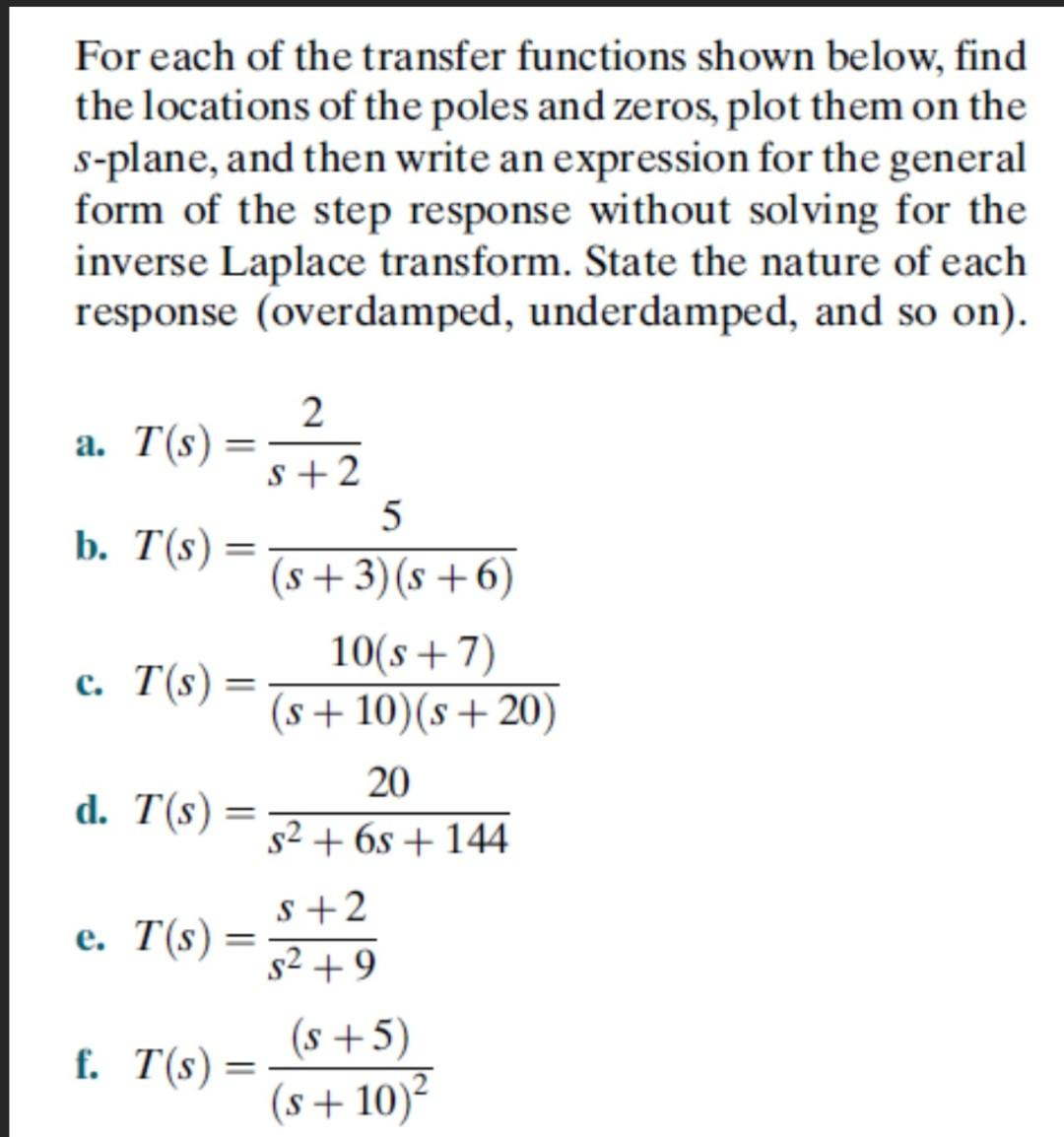# Question   For each of the transfer functions shown below, find the locations of the poles and zeros, plot them on the $$s$$-plane, and then write an expression for the general form of the step response without solving for the inverse Laplace transform. State the nature of each response (overdamped, underdamped, and so on). a. $$T(s)=\frac{2}{s+2}$$ b. $$T(s)=\frac{5}{(s+3)(s+6)}$$ c. $$T(s)=\frac{10(s+7)}{(s+10)(s+20)}$$ d. $$T(s)=\frac{20}{s^{2}+6 s+144}$$ e. $$T(s)=\frac{s+2}{s^{2}+9}$$ f. $$T(s)=\frac{(s+5)}{(s+10)^{2}}$$PV7ORI The Asker · Electrical EngineeringTranscribed Image Text: For each of the transfer functions shown below, find the locations of the poles and zeros, plot them on the $$s$$-plane, and then write an expression for the general form of the step response without solving for the inverse Laplace transform. State the nature of each response (overdamped, underdamped, and so on). a. $$T(s)=\frac{2}{s+2}$$ b. $$T(s)=\frac{5}{(s+3)(s+6)}$$ c. $$T(s)=\frac{10(s+7)}{(s+10)(s+20)}$$ d. $$T(s)=\frac{20}{s^{2}+6 s+144}$$ e. $$T(s)=\frac{s+2}{s^{2}+9}$$ f. $$T(s)=\frac{(s+5)}{(s+10)^{2}}$$
More
Transcribed Image Text: For each of the transfer functions shown below, find the locations of the poles and zeros, plot them on the $$s$$-plane, and then write an expression for the general form of the step response without solving for the inverse Laplace transform. State the nature of each response (overdamped, underdamped, and so on). a. $$T(s)=\frac{2}{s+2}$$ b. $$T(s)=\frac{5}{(s+3)(s+6)}$$ c. $$T(s)=\frac{10(s+7)}{(s+10)(s+20)}$$ d. $$T(s)=\frac{20}{s^{2}+6 s+144}$$ e. $$T(s)=\frac{s+2}{s^{2}+9}$$ f. $$T(s)=\frac{(s+5)}{(s+10)^{2}}$$